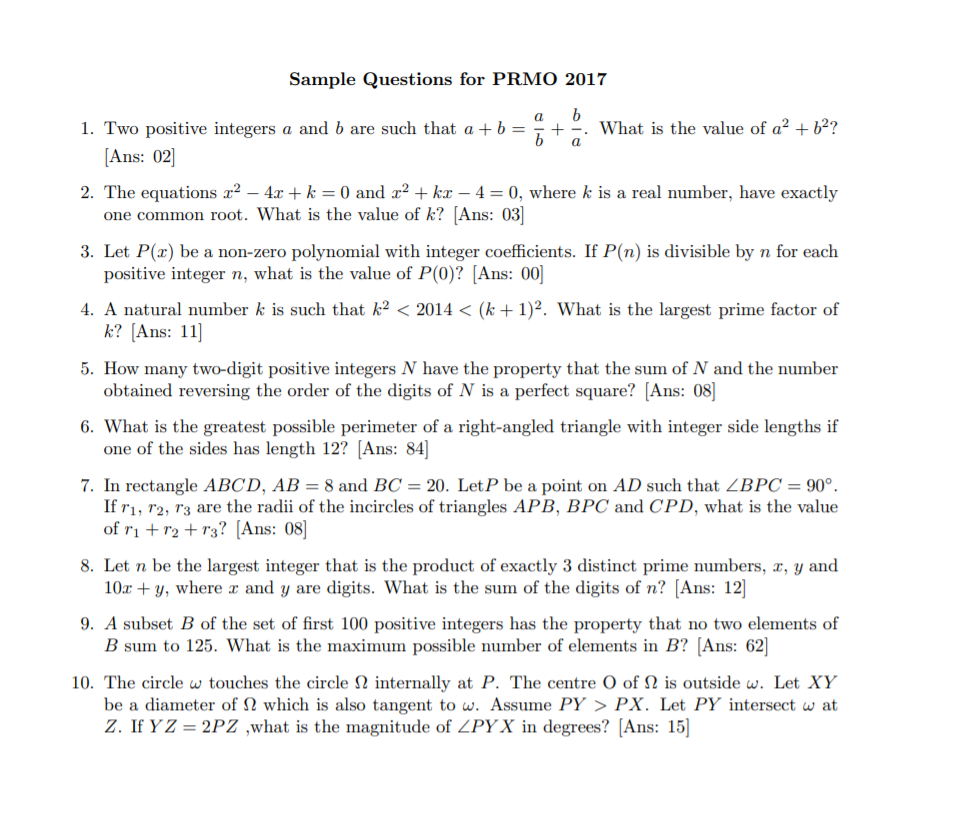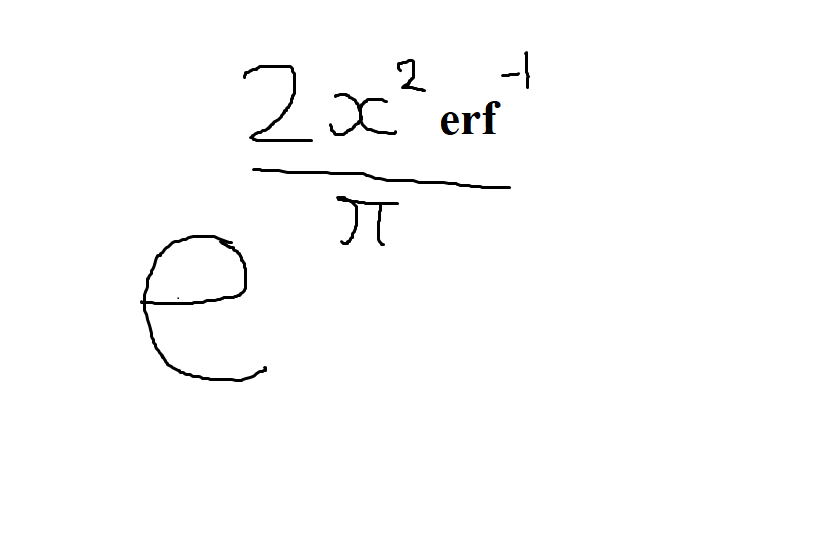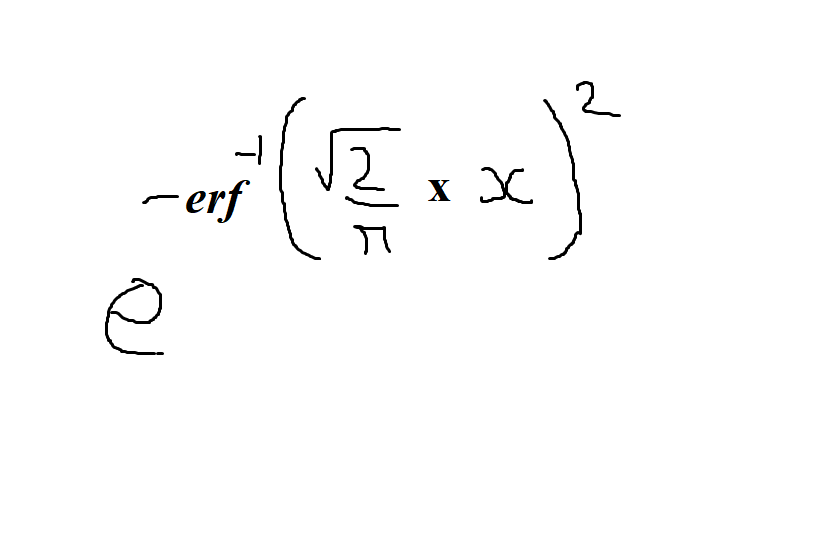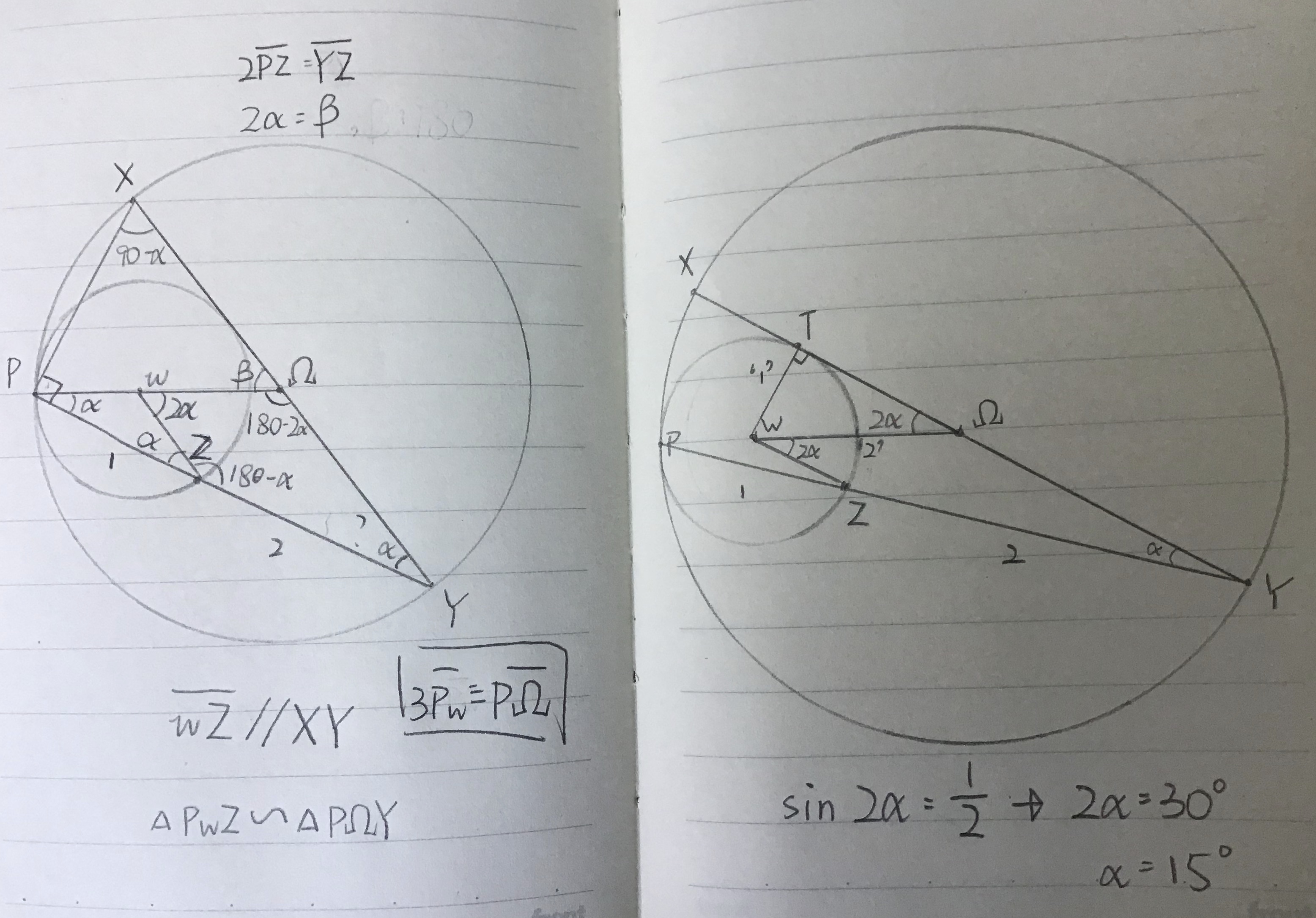# PRMO Sample Questions

Here are some Sample PRMO Questions, which Mahdi Raza gave me today on the Daily Challenges page.This can also be accessed here

I was able to solve some of them, but I wish for the solutions for the problems $3^{\text{rd}},6^{\text{th}},10^{\text{th}}$. I could not solve the $7^{\text{th}}$ one also, but he provided me a solution, so there is no need for it. Any help is appreciated! Thank you!Note by Vinayak Srivastava
6 months ago

This discussion board is a place to discuss our Daily Challenges and the math and science related to those challenges. Explanations are more than just a solution — they should explain the steps and thinking strategies that you used to obtain the solution. Comments should further the discussion of math and science.

When posting on Brilliant:

• Use the emojis to react to an explanation, whether you're congratulating a job well done , or just really confused .
• Ask specific questions about the challenge or the steps in somebody's explanation. Well-posed questions can add a lot to the discussion, but posting "I don't understand!" doesn't help anyone.
• Try to contribute something new to the discussion, whether it is an extension, generalization or other idea related to the challenge.

MarkdownAppears as
*italics* or _italics_ italics
**bold** or __bold__ bold
- bulleted- list
• bulleted
• list
1. numbered2. list
1. numbered
2. list
Note: you must add a full line of space before and after lists for them to show up correctly
paragraph 1paragraph 2

paragraph 1

paragraph 2

[example link](https://brilliant.org)example link
> This is a quote
This is a quote
    # I indented these lines
# 4 spaces, and now they show
# up as a code block.

print "hello world"
# I indented these lines
# 4 spaces, and now they show
# up as a code block.

print "hello world"
MathAppears as
Remember to wrap math in $$ ... $$ or $ ... $ to ensure proper formatting.
2 \times 3 $2 \times 3$
2^{34} $2^{34}$
a_{i-1} $a_{i-1}$
\frac{2}{3} $\frac{2}{3}$
\sqrt{2} $\sqrt{2}$
\sum_{i=1}^3 $\sum_{i=1}^3$
\sin \theta $\sin \theta$
\boxed{123} $\boxed{123}$

Sort by:

- 6 months ago

I have them before, I will try to give some solutions tomorrow if I will get time.

- 6 months ago

Also I have copied some of my questions from here.

- 6 months ago

Do you have more papers?

- 6 months ago

After completing these questions, if you need more then just tell me.

- 6 months ago

Thank you! I will surely check these!

- 6 months ago

- 6 months ago

Oh, I forgot this question! Thank you!

- 6 months ago

- 6 months ago

Ok, then I will post the solutions for the 3rd and 10th as @Zakir Husain has already posted them.

- 6 months ago

- 6 months ago

Problem 3's solution:

Let the polynomial P(x) = ${ a }_{ 0 }+{ a }_{ 1 }{ x }^{ 1 }+{ a }_{ 2 }{ x }^{ 2 }+...+{ a }_{ k }{ x }^{ k }$ where ${ a }_{ 0 },{ a }_{ 1 },{ a }_{ 2 },...,{ a }_{ k }{ x }^{ k }$ are the respective coefficients and $x$ is the variable.

So for P(n), x = n and thus P(n) = ${ a }_{ 0 }+{ a }_{ 1 }{ n }^{ 1 }+{ a }_{ 2 }{ n }^{ 2 }+...+{ a }_{ k }{ n }^{ k }$. Now we can factor out $n$ as common from the part of the expression leaving ${ a }_{ 0 }$ as $n({ a }_{ 1 }+{ a }_{ 2 }{ n }^{ 1 }+...+{ a }_{ k }{ n }^{ k-1 })$. Now obviously, this part is divisble by $n$ as $n$ is factored out.

But it is given that the whole expression P(n) is divisible by the positive integer $n$, which means ${ a }_{ 0 }$ also has to be divisible by any positive integer $n$ for the whole expression to be divisible. Now, ${ a }_{ 0 }$ could either be 0 or 1x2x3x4x...n (n = infinity as we are considering all positive integers) But if it is 1x2x3x4...xn it will be a number which does not have any definite value, as it divergers and infinity is not a number. So ${ a }_{ 0 }$ has to be 0.

Hence, now P(x) = ${ a }_{ 1 }{ x }^{ 1 }+{ a }_{ 2 }{ x }^{ 2 }+...+{ a }_{ k }{ x }^{ k }$ as ${ a }_{ 0 }$ = 0 so adding it to the polynomial would have no effect. Now we need to find P(0) so subtiuting x = 0, all the terms would be reduced to 0 as 0 raise to any power(leaving 0^0 which is still debtable) is 0 and 0 multiplied by any number is 0. Thus P(0) = 0+0+0+..+0 = 0.

- 6 months ago

@Vinayak Srivastava I might post the solution by tomorrow for the 10th as It is night so I might go to sleep.

- 6 months ago

No problem! Do you have more papers of this type?

- 6 months ago

Thank you for your solution! However, please write except 0 in the last line, as $0^0$ is not defined! :)

- 6 months ago

0^0 is debatable, some say 1 or some say undefined. So I will write the same thing there, you can check the wiki on Brilliant on this.

- 6 months ago

Yes, that is why I am asking you to add except 0 after "any number".

- 6 months ago

Oh, you have done it. Now it is good! :)

- 6 months ago

If at all anyone finds more of such questions, not just PRMO but maybe even harder, then please share :)

- 6 months ago

Check the Millennium Prize problems: Link

- 6 months ago

lol, really 😂

- 6 months ago

Solve the differential equation for $f(x)$ (find $f(x)$):

$f''(x)\times f(x)=1$

Note:

• $f''(x)$ is the second derivative of $f(x)$

• Clue : $f'(x)=\sqrt{2\ln(f(x))}$

- 6 months ago

I worked it out @Zakir Husain I think it will beif the constants after integrating are 0 as it suggests from your clue and erf is the Error function

- 6 months ago

I can't understand.

- 6 months ago

Check out now.

- 6 months ago

$e^{\dfrac{2x^2\times erf^{-1}(\red{???})}{\pi}}$

- 6 months ago

Oh sorryIt is $\sqrt { \frac { 2 }{ \pi } }$ there.

- 6 months ago

Is this correct? I had previously forgotten the inputs and simplified falsely.

- 6 months ago

The first one is very close, I've rechecked my calculations. $\large{e^{-({erf^{-1}(\sqrt{\frac{2}{\pi}}\times x)})^2}}$, clue : multiply a constant

- 6 months ago

is it $\large{e^{-({erf^{-1}(\sqrt{\frac{2}{\pi}}\times x)})^2}}$ or it is $\large{e^{-{erf^{-1}((\sqrt{{\frac{2}{\pi}}}\times x)^2)}}}$

- 6 months ago

The first one where the input is squared, there is also i which I forgot to put because my notebook page has become a mess, so I wrote the answer in a very scribbled way.

- 6 months ago

The first one is very close, I've rechecked my calculations. $\large{e^{-({erf^{-1}(\sqrt{\frac{2}{\pi}}\times x)})^2}}$, clue : multiply a constant

- 6 months ago

There is a -i (imaginary unit) in the input of the inverse of error function.

- 6 months ago

Yes, but it will be more better if it will be $\large{i\times e^{-({erf^{-1}(\sqrt{\frac{2}{\pi}}\times x)})^2}}$

- 6 months ago

Check the new problem

- 6 months ago

If it's $\large{e^{-({erf^{-1}(\sqrt{\frac{2}{\pi}}\times x)})^2}}$ then that is not the accurate answer, but it is very close to it!

- 6 months ago

Don’t wrap maths in text :)

- 6 months ago

Actually I was doing something else, I was adding a text and separating it.

- 6 months ago

Q. Simplify the complex term below, and don't answer in terms of trigonometric functions:

$\frac{\sqrt{1+\sqrt{3}i}}{\sqrt{2}}+\frac{\sqrt{1-\sqrt{3}i}}{\sqrt{2}}$

Where $i$ is the imaginary unit

- 6 months ago

2, since this is $w,w^2$, the solutions to $x^3=1$.

- 6 months ago

What $w,w^2$ and what $x^3$, I don't understand what are you saying. (this is cube root)

- 6 months ago

Oh! Whups, took it as cube :P

- 6 months ago

Yikes, this is $-w$ :P

- 6 months ago

#6
Since you want the maximum side length, 12 should be the shortest side in the triangle.
Possible arrangements:12-16-20(3-4-5), 5-12-13(seriously?),

12-35-37(easily missed, but break $12^2$ to $71+73$, then since 71 is the 36th odd number, $12^2+(36-1)^2=(36-1+2)^2$.)

- 6 months ago

- 6 months ago

I usually do solve the PRMO ones, when I can understand them, but I never solve the SAT ones, as I don't have to give it, and the questions are too tough for me.

- 6 months ago

Oh. But I think there is one in a few that’s easy enough :) just check the note ;)

- 6 months ago

@Jeff Giff what is your real age, 13?

- 6 months ago

Yup, and I am dead serious, trust me.

- 6 months ago

#10The ‘1’ and ‘2’ is derived from the fact that $3\overline{Pw} =\overline{P\Omega}$, derived from the fact that $\triangle PwZ\sim \triangle P\Omega Y$. Last bit was studying $\triangle wT\Omega$.
@Vinayak Srivastava

- 6 months ago

@Vinayak Srivastava, try this:

$OEE$
$\times EE$
$—$
$EOEE$
$EOE$
$—$
$OOEE$
The multiplication above has been replaced with the parity(even or odd) of its digits, whereas O stands for odd, E stands for even. For example, 84 would become EE, since both 8 and 4 are even, and 1257 would become OEOO according to this pattern.

- 6 months ago

- 6 months ago

oh. Nice translator!

- 6 months ago

@Vinayak Srivastava which grade are you?

- 5 months, 2 weeks ago